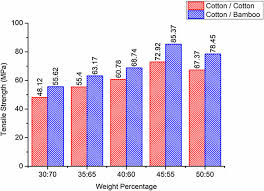# How to Calculate and Solve for Critical Fibre Strength for Composite | Composites

The critical fibre strength for composite is illustrated by the image below.To compute for critical fibre strength for composite, three essential parameters are needed and these parameters are Stress in Matrix at Fibre Failure (σ’m), Fibre Critical Tensile Strength (σ*f) and Volume Fraction of the Fibre (Vf).

The formula for calculating critical fibre strength for composite:

σ*a = σ’m(1 – Vf) + σ*fVf

Where:

σ*a = Critical Fibre Strength for Composite
σ’m = Stress in Matrix at Fibre Failure
σ*f = Fibre Critical Tensile Strength
Vf = Volume Fraction of the Fibre

Let’s solve an example;
Find the critical fibre strength for composite when the stress in matrix at fibre failure is 12, the fibre critical tensile strength is 10 and the volume fraction of the fibre is 9.

This implies that;

σ’m = Stress in Matrix at Fibre Failure = 12
σ*f = Fibre Critical Tensile Strength = 10
Vf = Volume Fraction of the Fibre = 9

σ*a = σ’m(1 – Vf) + σ*fVf
σ*a = 12(1 – 9) + (10)(9)
σ*a = 12(-8) + (90)
σ*a = (-96) + (90)
σ*a = -6

Therefore, the critical fibre strength for composite is -6 Pa.

Nickzom Calculator – The Calculator Encyclopedia is capable of calculating the critical fibre strength for composite.

To get the answer and workings of the critical fibre strength for composite using the Nickzom Calculator – The Calculator Encyclopedia. First, you need to obtain the app.

You can get this app via any of these means:

You can also try the demo version via https://www.nickzom.org/calculator

Apple (Paid) – https://itunes.apple.com/us/app/nickzom-calculator/id1331162702?mt=8
Once, you have obtained the calculator encyclopedia app, proceed to the Calculator Map, then click on Materials and Metallurgical under Engineering.Now, Click on Composites under Materials and MetallurgicalNow, Click on Critical Fibre Strength for Composite under CompositesThe screenshot below displays the page or activity to enter your values, to get the answer for the critical fibre strength for composite according to the respective parameter which is the Stress in Matrix at Fibre Failure (σ’m), Fibre Critical Tensile Strength (σ*f) and Volume Fraction of the Fibre (Vf).Now, enter the values appropriately and accordingly for the parameters as required by the Stress in Matrix at Fibre Failure (σ’m) is 12, Fibre Critical Tensile Strength (σ*fis 10 and Volume Fraction of the Fibre (Vf) is 9.Finally, Click on CalculateAs you can see from the screenshot above, Nickzom Calculator– The Calculator Encyclopedia solves for the critical fibre strength for composite and presents the formula, workings and steps too.